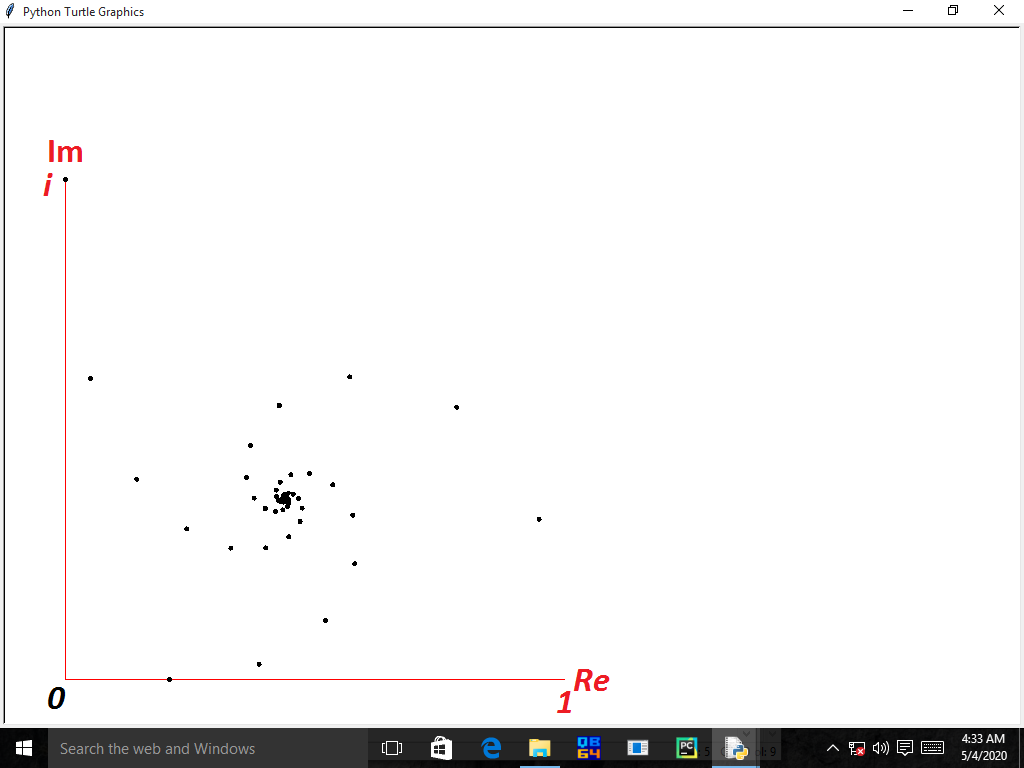# Fantastic Imaginary unit!

From Euler's identity : $e^{i\theta}=\cos(\theta)+i\sin(\theta)$ For $$\theta=\frac{1}{2}\pi$$ $e^{i\frac{1}{2}\pi}=i$ Let $$a=e^{\frac{1}{2}\pi}$$ $\Rightarrow a^i=i \Rightarrow a^{a^{a^{a^{...}}}}=i$ Now a question which I'm unable to solve and it is very interesting one! (see tetration if you aren't understanding ${^n i}$) $\lim_{n \to \infty}{^n i}=?$Note by Zakir Husain
1 year ago

This discussion board is a place to discuss our Daily Challenges and the math and science related to those challenges. Explanations are more than just a solution — they should explain the steps and thinking strategies that you used to obtain the solution. Comments should further the discussion of math and science.

When posting on Brilliant:

• Use the emojis to react to an explanation, whether you're congratulating a job well done , or just really confused .
• Ask specific questions about the challenge or the steps in somebody's explanation. Well-posed questions can add a lot to the discussion, but posting "I don't understand!" doesn't help anyone.
• Try to contribute something new to the discussion, whether it is an extension, generalization or other idea related to the challenge.
• Stay on topic — we're all here to learn more about math and science, not to hear about your favorite get-rich-quick scheme or current world events.

MarkdownAppears as
*italics* or _italics_ italics
**bold** or __bold__ bold
- bulleted- list
• bulleted
• list
1. numbered2. list
1. numbered
2. list
Note: you must add a full line of space before and after lists for them to show up correctly
paragraph 1paragraph 2

paragraph 1

paragraph 2

[example link](https://brilliant.org)example link
> This is a quote
This is a quote
    # I indented these lines
# 4 spaces, and now they show
# up as a code block.

print "hello world"
# I indented these lines
# 4 spaces, and now they show
# up as a code block.

print "hello world"
MathAppears as
Remember to wrap math in $$ ... $$ or $ ... $ to ensure proper formatting.
2 \times 3 $2 \times 3$
2^{34} $2^{34}$
a_{i-1} $a_{i-1}$
\frac{2}{3} $\frac{2}{3}$
\sqrt{2} $\sqrt{2}$
\sum_{i=1}^3 $\sum_{i=1}^3$
\sin \theta $\sin \theta$
\boxed{123} $\boxed{123}$

## Comments

Sort by:

Top Newest

I have plotted the graph of ${^ni}$ on the complex plain using python:The $\lim_{n \to \infty}{^ni}$ is converging in the darker middle region.

${^{960}i}\approx 0.43828...+0.36059...i$

- 1 year ago

Log in to reply

Let the limit be $L$. Does $i^L=L?$

- 1 year ago

Log in to reply

Yes

- 12 months ago

Log in to reply

BTW, I don’t really understand $n^i,$ although I do know tetration. Could you explain it to me? Thanks :)

- 1 year ago

Log in to reply

${^ni}=i^{i^{i^{i^{.^{.^{.}}}}}}$ where there are $n$ $i's$

- 1 year ago

Log in to reply

Well that I know... :) but $i^i$ I do not understand :D $i$ times itself $i$ times?

- 1 year ago

Log in to reply

See how we evaluate $i^i$:

From Euler's identity (It comes every place you talk about $i$): $e^{i\theta}=\cos(\theta)+i\sin(\theta)$ At $\theta = \frac{1}{2}\pi$ $e^{i\frac{1}{2}\pi}=i$ Raising both sides to $i$ $(e^{i\frac{1}{2}\pi})^i=i^i$ $i^i=(e^{i\frac{1}{2}\pi})^i=e^{\red{i}\frac{1}{2}\pi \red{i}}=e^{-\frac{1}{2}\pi}$

- 1 year ago

Log in to reply

Oic. Thank you :)

- 1 year ago

Log in to reply

- 1 year ago

Log in to reply

Sorry but I can't be any help here since I don't know Calculus that well (I have just started the Fundamentals Course)

- 1 year ago

Log in to reply

I don't know tetration , but it seems you must calculate exponents from top to botom . Tower of "a" you equal to "i" is right if at the end of tower is and "i" . To calculate the tower you must consider where braquets are . See tetration explanation.

- 12 months ago

Log in to reply

Tetration of a complex number to a infinite height can be done using the Lambert W function. The formula is W(-log(z))/(-log(z)). Here log(i)=iπ/2 and the formula gives the value that Zakir Husain has calculated.

- 12 months ago

Log in to reply

×

Problem Loading...

Note Loading...

Set Loading...